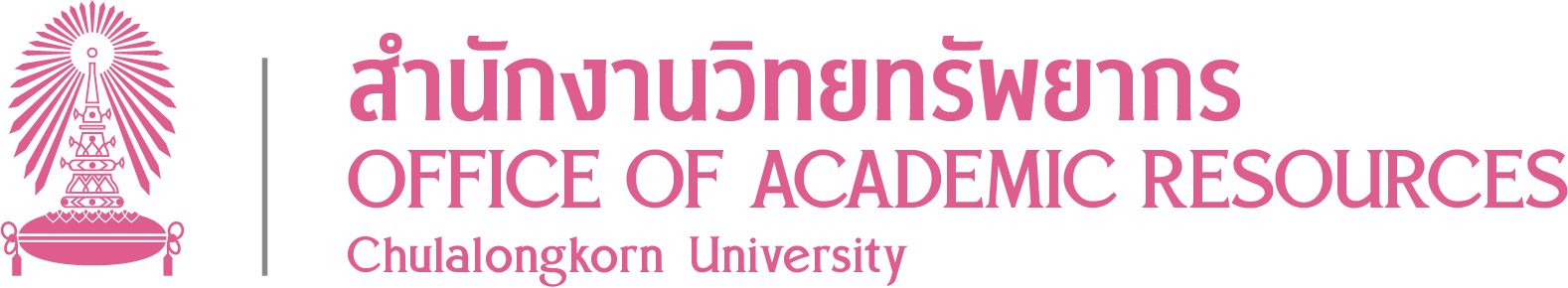Author Khuri, Andrใae I., 1940- Linear model methodology [electronic resource] / Andrใae I. Khuri Boca Raton : CRC Press, c2010 http://marc.crcnetbase.com/isbn/9781420010442 xix, 542 p. : ill

CONTENT

Linear models : some historical perspectives -- Basic elements of linear algebra -- Basic concepts in matrix algebra -- The multivariate normal distribution -- Quadratic forms in normal variables -- Full-rank linear models -- Less-than-full-rank linear models -- Balanced linear models -- The adequacy of Satterthwaite's approximation -- Unbalanced fixed-effects models -- Unbalanced random and mixed models -- Additional topics in linear models -- Generalized linear models

Linear models (Statistics) -- Textbooks Electronic books.